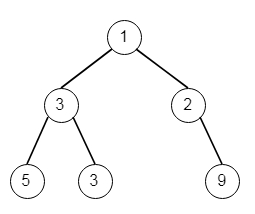# Maximum Width of Binary Tree in C++

Suppose we have a binary tree, we have to define a function to get the maximum width of the given tree. Here the width of a tree is the maximum width among all levels. We will consider the binary tree has the same structure as a full binary tree, but some nodes are null. The width of one level is actually the length between the end-nodes (the leftmost and right most non-null nodes in the level, where the null nodes between the end-nodes are also counted for the length calculation). So if the tree is like −Then the maximum width is 4, as the nodes of the last layer is [5,3,null,9]

To solve this, we will follow these steps −

• ans := 1, size := 0

• define a double ended queue q where we will store (node, value) pair.

• insert (root, 1) into q

• while q is not empty

• size := size of the q

• define a (node, value) pair curr

• if size is 1, then (node of the front element, 1) into q, delete element from q

• while size is not 0

• curr := front element of q, delete the front element from q

• if left of the curr node is not null, then

• create (left of the current node, 2*value of the curr) and insert into q

• if right of the curr node is not null, then

• create (right of the current node, 2*value of the curr + 1) and insert into q

• if size of q > 1, then

• ans := max of ans, value of the last element in q – value of first element of q + 1

• size := size – 1

• return ans

Let us see the following implementation to get better understanding −

## Example

Live Demo

#include <bits/stdc++.h>
using namespace std;
class TreeNode{
public:
int val;
TreeNode *left, *right;
TreeNode(int data){
val = data;
left = NULL;
right = NULL;
}
};
void insert(TreeNode **root, int val){
queue<TreeNode*> q;
q.push(*root);
while(q.size()){
TreeNode *temp = q.front();
q.pop();
if(!temp->left){
if(val != NULL)
temp->left = new TreeNode(val);
else
temp->left = new TreeNode(0);
return;
}else{
q.push(temp->left);
}
if(!temp->right){
if(val != NULL)
temp->right = new TreeNode(val);
else
temp->right = new TreeNode(0);
return;
}else{
q.push(temp->right);
}
}
}
TreeNode *make_tree(vector<int> v){
TreeNode *root = new TreeNode(v);
for(int i = 1; i<v.size(); i++){
insert(&root, v[i]);
}
return root;
}
class Solution {
public:
int widthOfBinaryTree(TreeNode* root) {
int ans = 0;
deque < pair <TreeNode*, int> > q;
q.push_back({root,1});
ans = 1;
int size;
while(!q.empty()){
size = q.size();
pair <TreeNode*, int> curr;
if(size == 1){
q.push_back({q.front().first, 1});
q.pop_front();
}
while(size--){
curr = q.front();
q.pop_front();
if(curr.first->left){
q.push_back({curr.first->left, 2 * curr.second});
}
if(curr.first->right){
q.push_back({curr.first->right, 2 * curr.second + 1});
}
}
if(q.size() > 1)
ans = max(ans, q.back().second - q.front().second + 1);
}
return ans;
}
};
main(){
vector<int> v = {1,3,2,5,3,NULL,9};
TreeNode *root = make_tree(v);
Solution ob;
cout << (ob.widthOfBinaryTree(root));
}

## Input

[1,3,2,5,3,null,9]

## Output

4